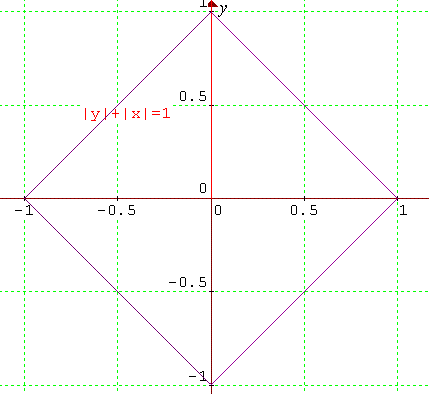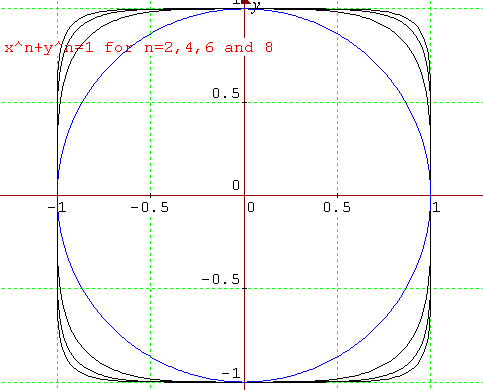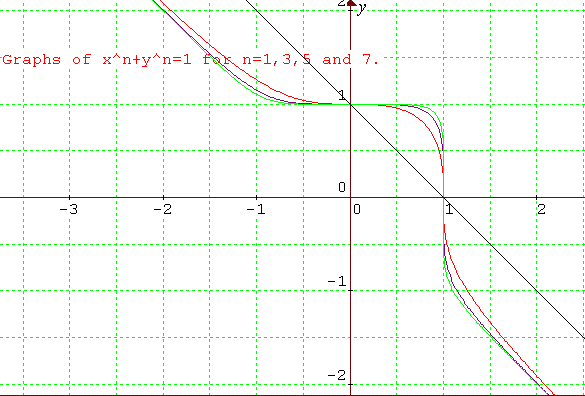#### You may also like### Degree Ceremony

What does Pythagoras' Theorem tell you about these angles: 90Â°, (45+x)Â° and (45-x)Â° in a triangle?### After Thought

Which is larger cos(sin x) or sin(cos x) ? Does this depend on x ?### Small Steps

Two problems about infinite processes where smaller and smaller steps are taken and you have to discover what happens in the limit.

# Squareness

##### Age 16 to 18Challenge LevelThis solution was submitted by Curt from Reigate College. Well done Curt.

(1) Explain the features of the graph of the relation $|x|+|y|=1.$

If the above equation is true then neither $|x|$ nor $|y|$ can be greater than 1. If say $1+h =|x| > 1$, we have: $1+h + |y| =1$ and so $|y| = -h < 0$, a contradiction, as $|a|\geq 0$ for any $a$. Therefore, this function is not defined for values of $x$ and $y$ greater than 1.

I now quote without proof the fact that if $|a| = t$ then $a =t$ or $a= -t$. This is useful when applied in this problem.

If $|y| = 1- |x|$ then $y = 1 - |x|$ or $y = -1 + |x|$. If $y = 1- |x|$ then $y= 1 + x$ or $y= 1-x$. Similarly, if $y = -1 + |x|$, then $y=x-1$ or $y=-x-1$.

The graph is the line segments (where $-1< x< 1$ and $-1< y< 1$) given by: $y=1-x$ in the first quadrant; $y=1+x$ in the second quadrant; $y=-1-x$ in the third quadrant and $y=x-1$ in the fourth quadrant.

Editor's note: By symmetry if $(a,b)$ satisfies this relation then so does $$(b,a),\ (-a,b)\ (-b,a)\ (-a,-b),\ (-b,-a),\ (a,-b),\ {\rm and}\ (b,-a)$$ so the graph is the square consisting of the four line segments described.

The line segments are perpendicular and intersect at (0,1), (-1,0) (0,-1) and (1,0). The square shape with vertices at the above co-ordinates, has symmetry in $y=x$, $y= -x$, $y=0$ and $x=0$. I refer to this as a graph, not a function, due to one-to-many relation.

2) As $n/(n+1)$ can be written as $1/(1+1/n)$, by dividing both numerator and denominator by $n$ we consider: \eqalign{ \left(1+{1\over n}\right)^n &= 1 + n\left({1\over n}\right) + {n(n-1)\over 2}\left({1\over n}\right)^2 + \ldots \cr &= 2 + \ldots \geq 2 > 1 .} Taking the nth root $$1+ {1\over n} \geq 2^{1/n} > 1^{1/n} =1$$ Hence $${1\over(1+1/n)}={n\over(n+1)} \leq {1\over 2^{1/n}} < 1.$$

(3) Taking the simple case $x^2 + y^2 = 1$ (the circle), and considering the first quadrant, it will be shown that $y$ decreases as $x$ increases. As this is in the first quadrant, the positive root alone is considered: $y = (1-x^2)^{1/2}.$ As $x$ increases to a maximum value of 1 on the interval $0 \leq x \leq 1$ so $x^2$ increases monotonically and $f(x)=1-x^2$ decreases monotonically (to confirm, check f'(x)=-2x).

If we have $f(x_1)> f(x_2)$ (with $x_1< x_2$), then taking the positive square root of both sides, we have ${f(x_1)}^{1/2} > {f(x_2)}^{1/2}$. So as $x$ increases, $y$ decreases.

This same method could be extended to all values of $n$. For $g(x) = 1-x^n$ then $g(x+h)< g(x)$ for $h> 0$ because $x^n$ increases monotonically on the interval $0< x< 1$. Clearly, if $x_1< x_2$ then $x_1^n< x_2^n$ so $1-x_1^n> 1-x_2^n$ and hence $g(x_1)> g(x_2)$. Taking the positive nth root of both sides, we get: $y_1 = {g(x_1)}^{1/n}> {g(x_2)}^{1/n}=y_2$. So $y_1 > y_2$ for $x_1 < x_2$ ; hence as $x$ increases in the first quadrant, $y$ decreases.

Editor's note: Alternatively we can use calculus to prove this. Fixing one value of $n$ in $x^n+y^n=1$: \eqalign{ y^n &= 1-x^n \cr ny^{n-1}{dy\over dx} &= -nx^{n-1}\cr {dy\over dx}&= -{x^{n-1}\over y^{n-1}} < 0.} As $x$ and $y$ are positive in the first quadrant we see that the derivative is negative which shows that $y$ decreases as $x$ increases.

When $x=y$, on the general graph $x^n + y^n = 1$ we get $2x^n=1$ so $x=y=1/(2^{1/n})$. From earlier in this question, we have for $n> 1$, $${n\over n+1} < {1\over 2^{1/n}}$$ so $x=y > n/(n+1)$. Therefore, as there is only one solution to $2x^n = 1$ in the first quadrant, the graph should lie outside the square with coordinates: $$(0,0),\ (0, n/(n+1)),\ (n/(n+1), n/(n+1)),\ (n/(n+1),0)$$ In the first quadrant, as $x,y\geq 0$, and on $x^n + y^n = 1$, neither $x$ nor $y$ may be greater than 1, there are no co-ordinates on the curve for which $x,y> 1$. If $x> 1$ then $x^n=1+g$, for $g> 0$ so $y^n=-g$, which is not allowed in the first quadrant. The curve must lie inside (or on at the co-ordinate axes) the square with coordinates (1,0), (0,1), (1,1), (0,0) NB, the curve will touch the square only at vertices (1,0) and (0,1).(4) As $n \to \infty$, $1/(1+1/n) = n/(1+n) \to 1$.

The square which the curve must lie within is fixed (as above). However, as $n$ increases, the square which the curve must lie outside of gets increasingly large. As the curve is squeezed between two squares, its shape becomes increasingly more squared.

For curves where $n$ is even, the curve becomes squeezed in all four quadrants because, by symmetry (exactly as in part (1) of this question) the graph is symmetric about $y=x$, $y=-x$, $y=0$ and about $x=0$.(5) In the first quadrant the graph of the relation $x^3 + y^3 = 1$ behaves similarly to other graphs where $n$ is even. However, the graph cannot be defined in the third quadrant for $x< 0$ and $y < 0$, for that would lead to the sum of two negative numbers being 1, which is not possible. The function is also defined for $x> 1$, for negative numbers do have real cube roots. If $x^3 = 1+a$ then $y^3 = -a$ and so $y=-a^{1/3}$ and $x = (1+a)^{1/3}$. For large $x$ (large $a$) this shows that $x \approx -y$, therefore the graph reaches an asymptote with $y= -x$. The same thing happens as $x \to -\infty$ in the second quadrant.

The same features occur for the whole family of graphs of $x^n + y^n=1$ for odd $n$.

The graphs of $|x^n| + |y^n| = 1$ for odd $n$ would have the symmetrical squarish shape, reflecting the family of graphs in the first quadrant into the remaining quadrants (see part 1).